|

# 百得发布新品牌战略计划 代言人范冰冰惊艳亮相

3月29日，中国知名厨卫品牌百得厨卫在中国广州召开主题为“凌越巅峰，世界百得”的品牌发布会。会上，百得正式向全球发布其品牌战略升级计划，并强势发布百得系列新品——新一代隐形智能烟机幻影T768以及新一代高热效燃气灶劲焱Q988两款新品。百得品牌形象代言人范冰冰盛装出席，为百得品牌升级和新品上市助阵。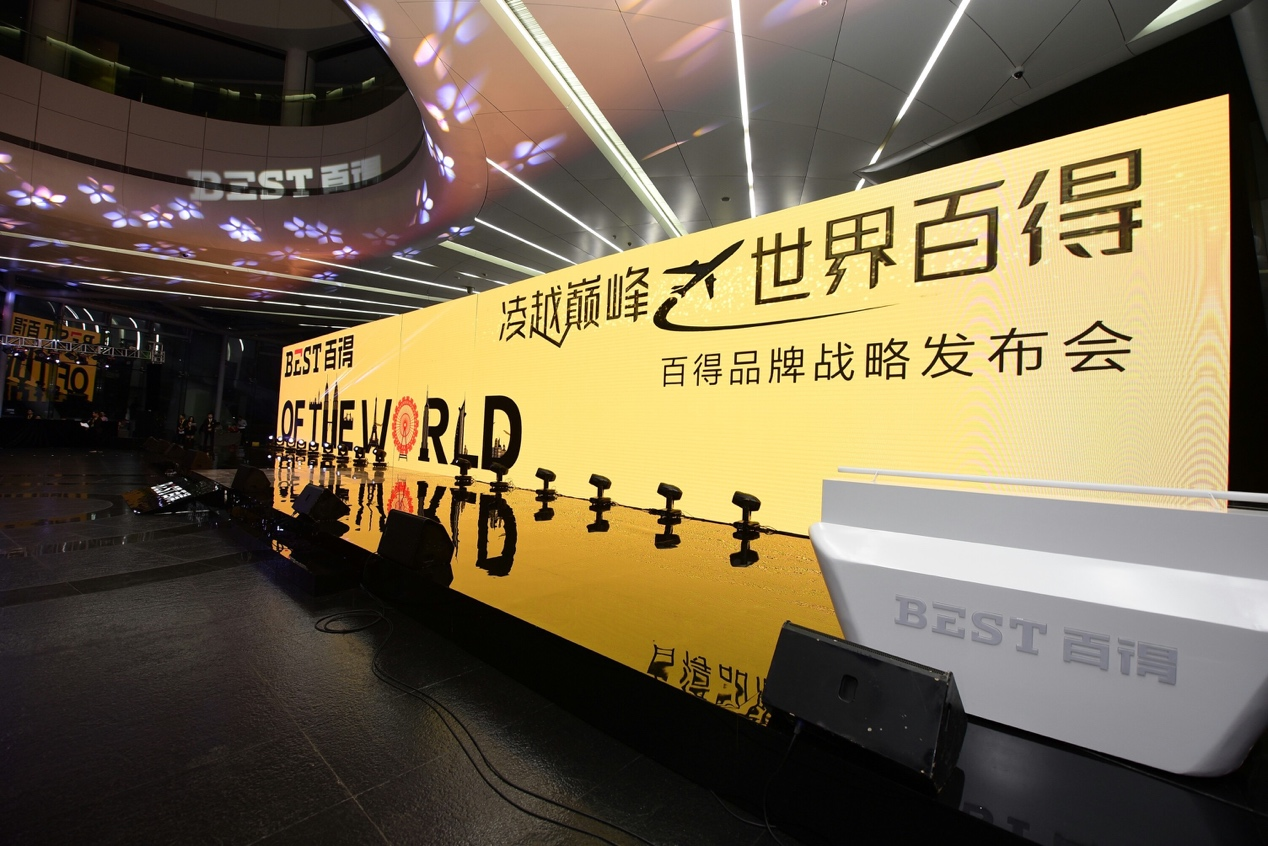这是百得首次把“全球专业厨卫”这一品牌定位提升至战略高度，正式吹响了百得品牌战略迭代升级的号角，预示着百得将全力开辟一条通向更广阔厨卫市场的道路。

百得品牌战略发布会盛况空前，巨星范冰冰登场助阵

本次发布会吸引了近百名媒体、行业人士参与，可谓是2018年度厨卫行业的盛宴。发布会现场分为外场和内场两部分，外场设有巨型签到墙和世界厨房体验区以及互动拍照区三大板块。在世界厨房体验区，不同国家的厨房情景区中集中展示了百得的全系产品，内场更设有最新产品展示和体验区，让所有参会嘉宾都可以零距离接触最新智能产品。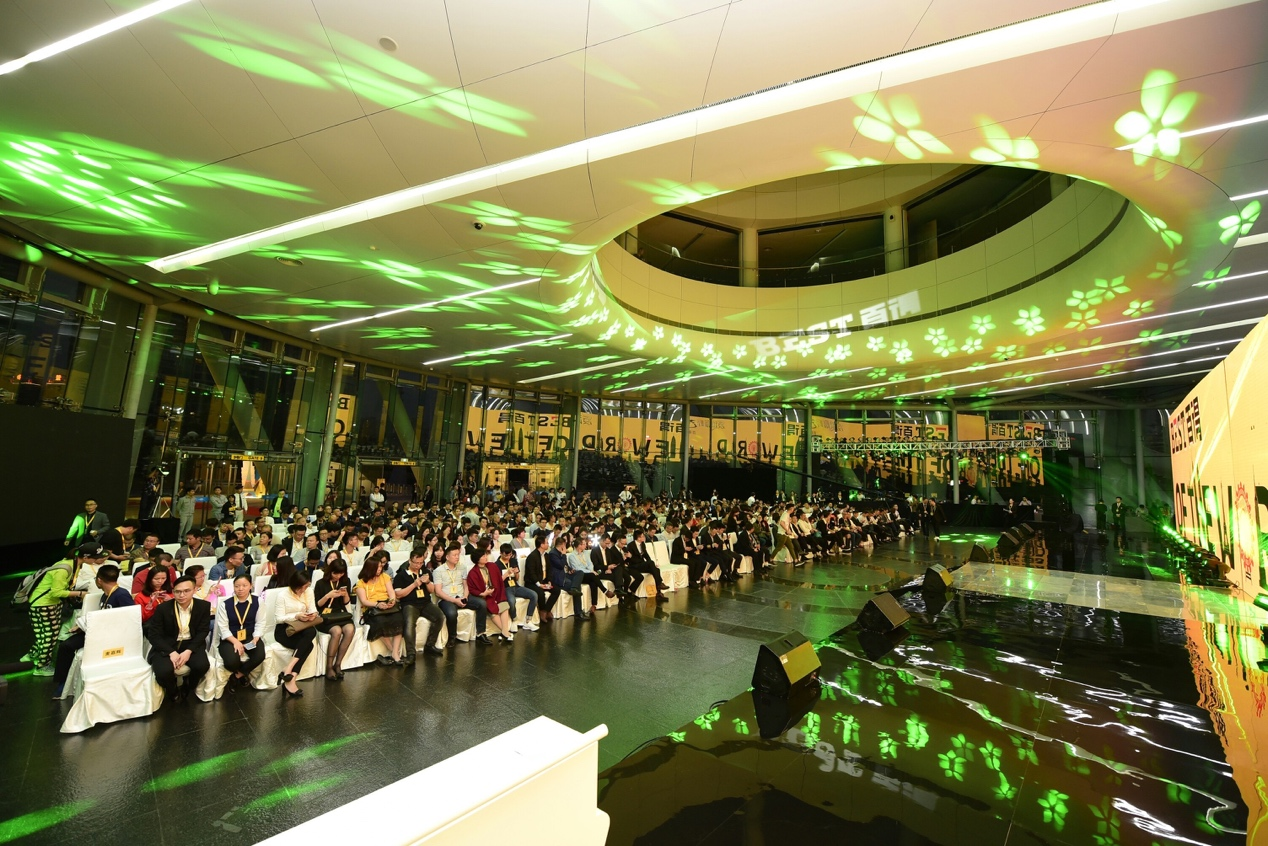会上，百得厨卫总经理潘垣枝在肯定百得近年来取得的优异成绩基础上，宣布百得品牌发展新战略方向——打造“全球专业厨卫”品牌， 提出新的品牌主张——“126个国家的选择”。同时，发布会上潘总还公布了百得新品牌LOGO。新LOGO体现了百得在全球126个国家取得的成就与进步，更加清晰地传递出百得“全球专业厨卫”：专业、品质、实用、创新的品牌核心价值。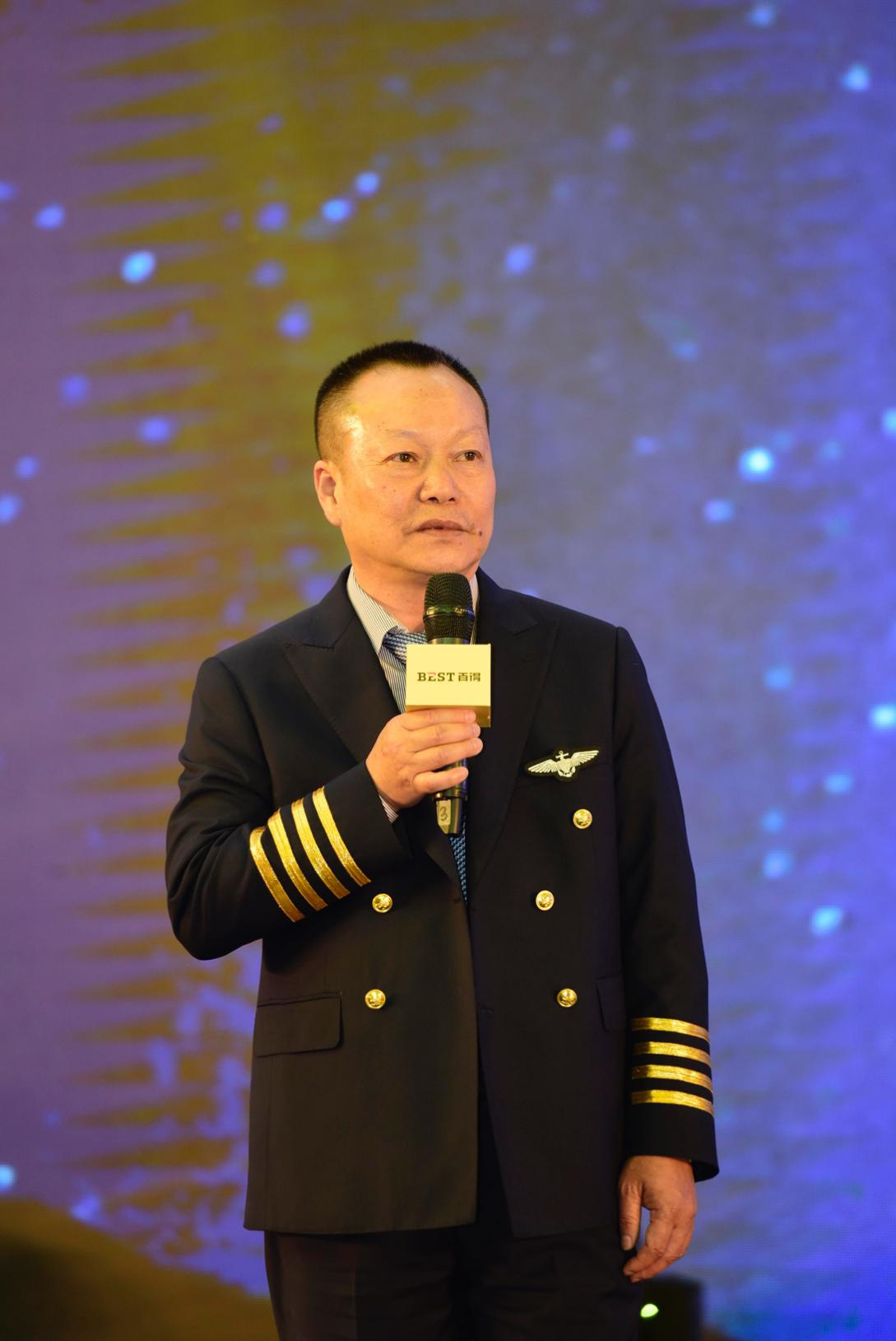此次发布会中，百得还隆重推出两款最新产品，分别是新一代隐形智能烟机幻影T768以及新一代高热效燃气灶劲焱Q988。新一代隐形智能烟机幻影T768拥有15项国家专利，兼有iWave 手势智控、iClean 智清洁以及独特宽屏照明灯和磁吸式集油杯四大产品特色，更有星空黑和幻彩金两种颜色可供消费者选择。无论从功能上和外观上，都势必吸引众多消费者的青睐。而新一代高热效燃气灶劲焱Q988，除了拥有涡喷式燃烧技术专利外，还拥有劲焱梯旋火，热效率高达67%，优于行业一级能效，顺应新时代环保节能的消费观念，可以说是现代家庭人群的最佳选择。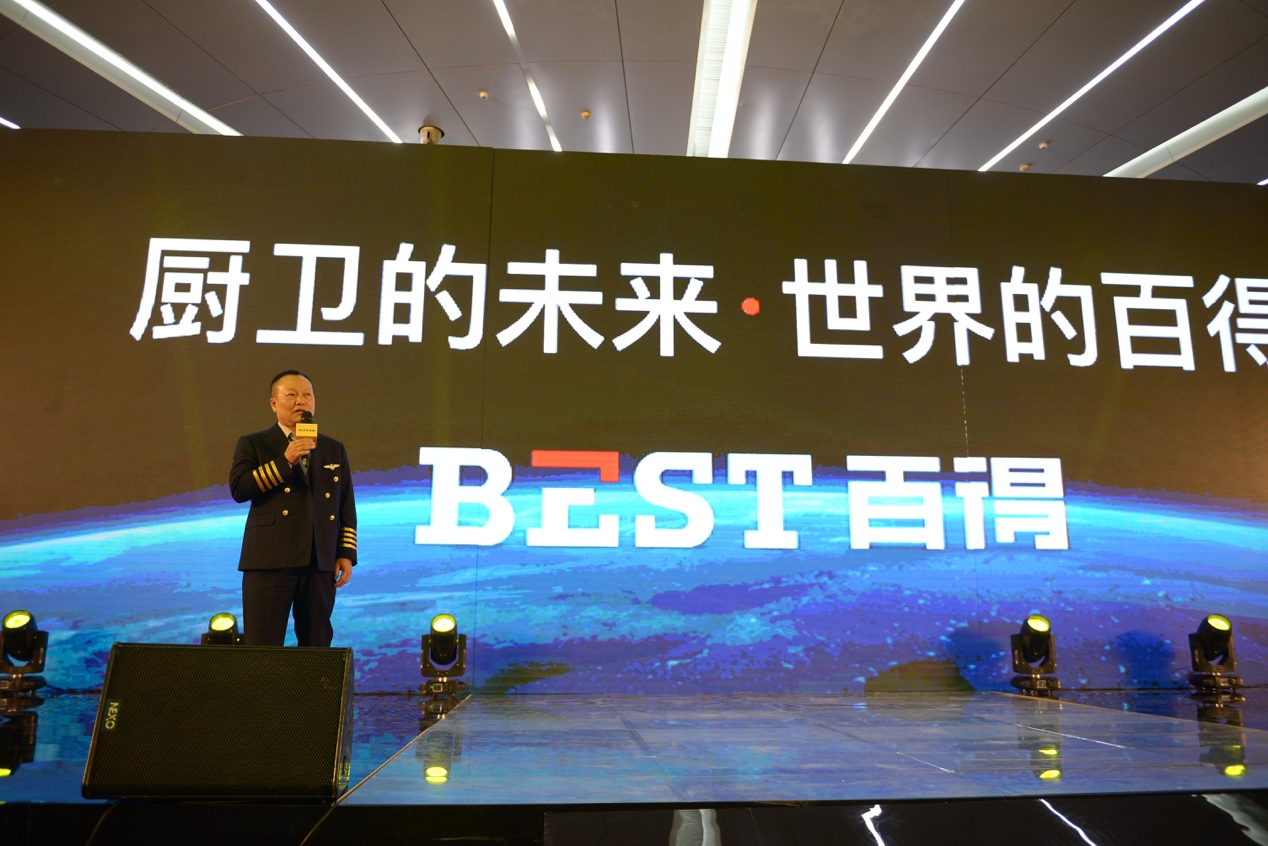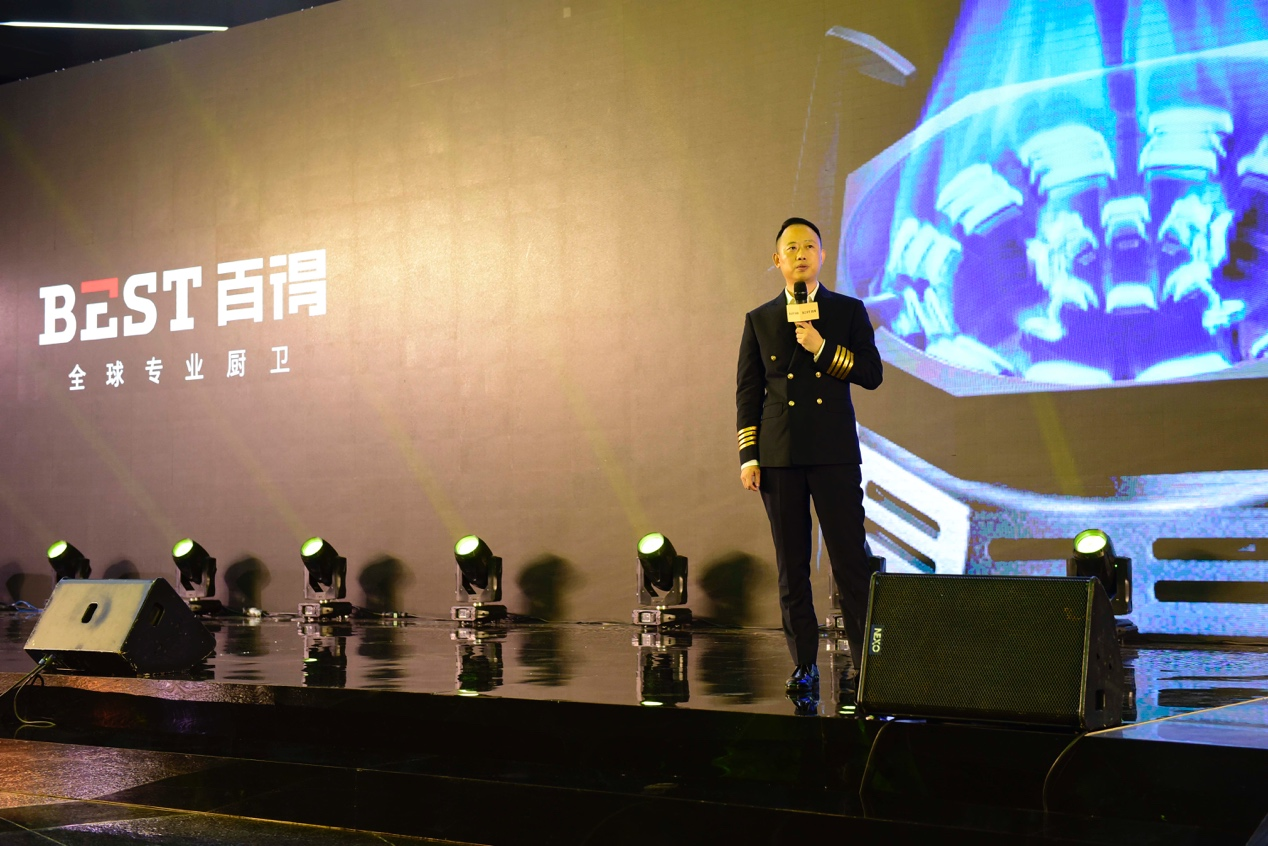如果说百得品牌战略和新产品的亮相满足了大家的好奇心，那么百得品牌形象代言人范冰冰的惊艳出场更是掀起了媒体关注的高潮。范冰冰一袭盛装出场，亲自上台参与互动体验，为新产品性能和设计点赞，并接受百得品牌授予的“百得首席生活官”称号。范冰冰表示非常认同百得的新品牌定位，对百得未来的发展前景看好。可以预见的是，凭借范冰冰的自身号召力以及话题传播力，百得将进一步提升品牌的知名度和曝光度。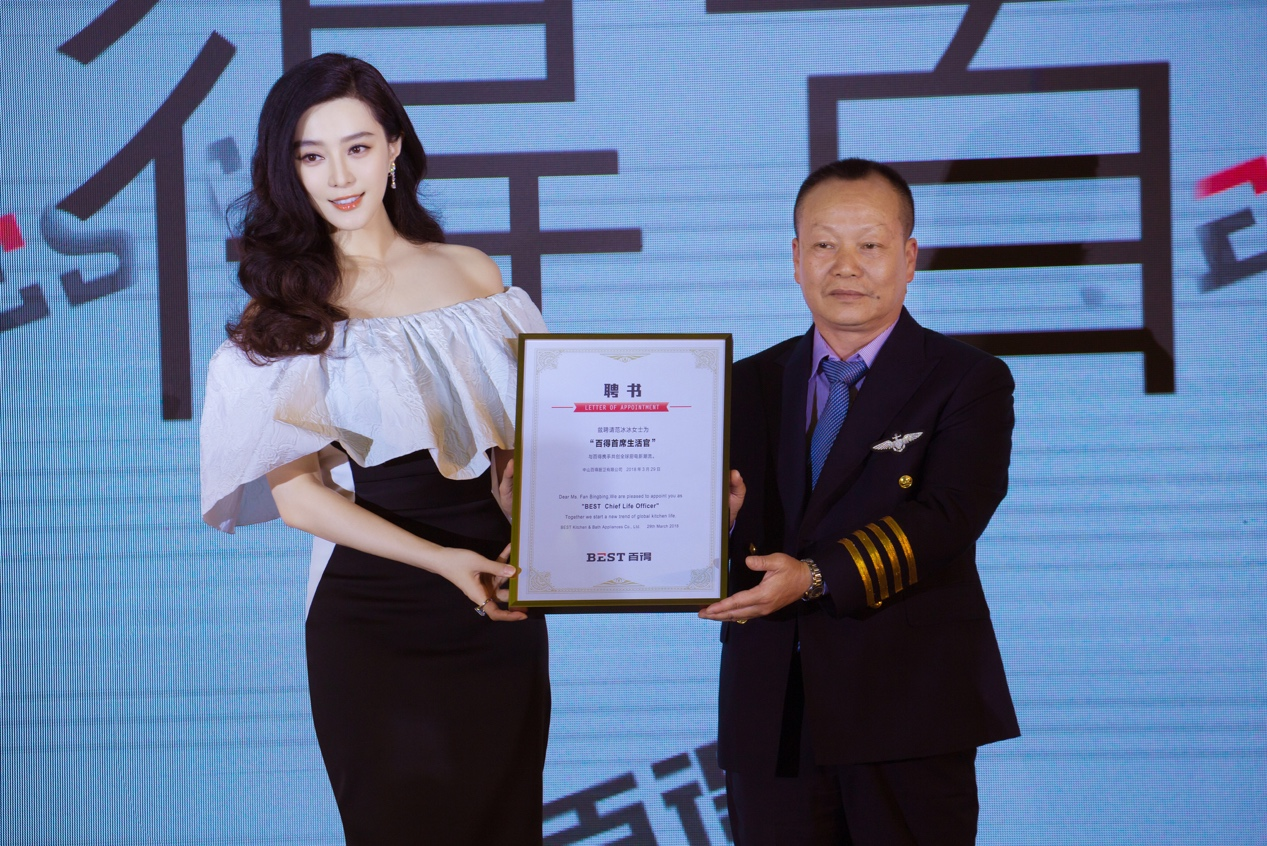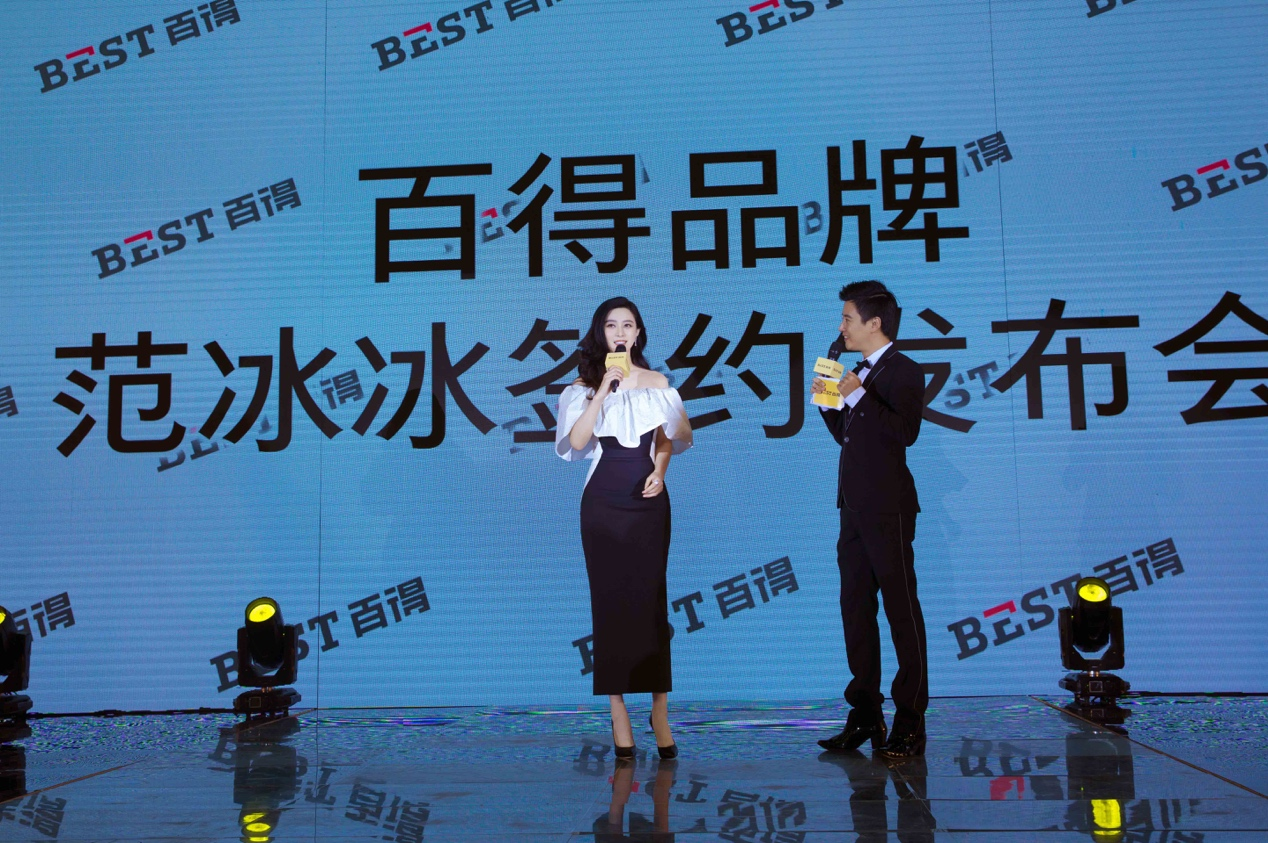启动品牌全新升级，百得抢滩全球厨卫市场

近年来中国厨卫市场进入“快车道”。家电产业发展重心正从客厅经济向厨卫经济转移。然而，随着全球家电市场发展进入新时代，中国厨卫行业面临着新环境、新问题、新机遇，转型升级更需要新力量。以百得为代表的中国厨卫企业，正告别以往追求降低成本和扩大规模的经营方式。百得不断通过产品和品牌升级，进一步增强企业发展创新能力，力求占据全球市场优势。

对于目前已经牢牢占据城镇中层阶层厨卫消费市场的百得而言，此次品牌发布会可以说是顺应现代全球化市场发展的必由之路。从国内市场走向国际市场，放眼世界，逐鹿全球，这是每一个具有全球视野的大国品牌应该具有的历史使命和企业责任，百得当然毫不例外。

另一方面，目前国内厨卫市场竞争趋于白热化，各大主流品牌已牢据一二线城市消费市场。如果想要突破和占领更多的市场份额，除了提升自身产品实力外，百得整体品牌战略大升级已是势在必行。百得正是抓住这一契机，重磅出击，率先签约范冰冰，同时通过本次品牌发布会，向世界宣布全新品牌升级和改造，进一步增强品牌知名度和美誉度，势必成为城镇中产阶层消费者的首选品牌。与此同时，百得品牌战略升级计划也是对华帝集团3年冲击100亿目标的强势呼应，为华帝集团最终实现这一宏伟目标夯实基础。“百得不仅是在布局当下，更是在布局未来。” 百得厨卫总经理潘垣枝表示，百得率先通过品牌迭代升级谋划未来，势必会进一步增强行业及消费者对百得品牌的关注度，同时对于百得未来品牌发展之路，更有举足轻重的意义。此次品牌战略升级计划，必将进一步扩大百得潜在消费市场，带动中国厨电品牌走出国门，为民族科技企业构筑更强信心和崛起势能。可以预见，未来百得品牌必将拥有更为广阔的发展前景和更大的市场份额。

`声明：本文由入驻焦点开放平台的作者撰写，除焦点官方账号外，观点仅代表作者本人，不代表焦点立场错误信息举报电话： 400-099-0099，邮箱：jubao@vip.sohu.com，或点此进行意见反馈，或点此进行举报投诉。`A B C D E F G H J K L M N P Q R S T W X Y Z
A - B - C - D - E
• A
• 鞍山
• 安庆
• 安阳
• 安顺
• 安康
• 澳门
• B
• 北京
• 保定
• 包头
• 巴彦淖尔
• 本溪
• 蚌埠
• 亳州
• 滨州
• 北海
• 百色
• 巴中
• 毕节
• 保山
• 宝鸡
• 白银
• 巴州
• C
• 承德
• 沧州
• 长治
• 赤峰
• 朝阳
• 长春
• 常州
• 滁州
• 池州
• 长沙
• 常德
• 郴州
• 潮州
• 崇左
• 重庆
• 成都
• 楚雄
• 昌都
• 慈溪
• 常熟
• D
• 大同
• 大连
• 丹东
• 大庆
• 东营
• 德州
• 东莞
• 德阳
• 达州
• 大理
• 德宏
• 定西
• 儋州
• 东平
• E
• 鄂尔多斯
• 鄂州
• 恩施
F - G - H - I - J
• F
• 抚顺
• 阜新
• 阜阳
• 福州
• 抚州
• 佛山
• 防城港
• G
• 赣州
• 广州
• 桂林
• 贵港
• 广元
• 广安
• 贵阳
• 固原
• H
• 邯郸
• 衡水
• 呼和浩特
• 呼伦贝尔
• 葫芦岛
• 哈尔滨
• 黑河
• 淮安
• 杭州
• 湖州
• 合肥
• 淮南
• 淮北
• 黄山
• 菏泽
• 鹤壁
• 黄石
• 黄冈
• 衡阳
• 怀化
• 惠州
• 河源
• 贺州
• 河池
• 海口
• 红河
• 汉中
• 海东
• 怀来
• I
• J
• 晋中
• 锦州
• 吉林
• 鸡西
• 佳木斯
• 嘉兴
• 金华
• 景德镇
• 九江
• 吉安
• 济南
• 济宁
• 焦作
• 荆门
• 荆州
• 江门
• 揭阳
• 金昌
• 酒泉
• 嘉峪关
K - L - M - N - P
• K
• 开封
• 昆明
• 昆山
• L
• 廊坊
• 临汾
• 辽阳
• 连云港
• 丽水
• 六安
• 龙岩
• 莱芜
• 临沂
• 聊城
• 洛阳
• 漯河
• 娄底
• 柳州
• 来宾
• 泸州
• 乐山
• 六盘水
• 丽江
• 临沧
• 拉萨
• 林芝
• 兰州
• 陇南
• M
• 牡丹江
• 马鞍山
• 茂名
• 梅州
• 绵阳
• 眉山
• N
• 南京
• 南通
• 宁波
• 南平
• 宁德
• 南昌
• 南阳
• 南宁
• 内江
• 南充
• P
• 盘锦
• 莆田
• 平顶山
• 濮阳
• 攀枝花
• 普洱
• 平凉
Q - R - S - T - W
• Q
• 秦皇岛
• 齐齐哈尔
• 衢州
• 泉州
• 青岛
• 清远
• 钦州
• 黔南
• 曲靖
• 庆阳
• R
• 日照
• 日喀则
• S
• 石家庄
• 沈阳
• 双鸭山
• 绥化
• 上海
• 苏州
• 宿迁
• 绍兴
• 宿州
• 三明
• 上饶
• 三门峡
• 商丘
• 十堰
• 随州
• 邵阳
• 韶关
• 深圳
• 汕头
• 汕尾
• 三亚
• 三沙
• 遂宁
• 山南
• 商洛
• 石嘴山
• T
• 天津
• 唐山
• 太原
• 通辽
• 铁岭
• 泰州
• 台州
• 铜陵
• 泰安
• 铜仁
• 铜川
• 天水
• 天门
• W
• 乌海
• 乌兰察布
• 无锡
• 温州
• 芜湖
• 潍坊
• 威海
• 武汉
• 梧州
• 渭南
• 武威
• 吴忠
• 乌鲁木齐
X - Y - Z
• X
• 邢台
• 徐州
• 宣城
• 厦门
• 新乡
• 许昌
• 信阳
• 襄阳
• 孝感
• 咸宁
• 湘潭
• 湘西
• 西双版纳
• 西安
• 咸阳
• 西宁
• 仙桃
• 西昌
• Y
• 运城
• 营口
• 盐城
• 扬州
• 鹰潭
• 宜春
• 烟台
• 宜昌
• 岳阳
• 益阳
• 永州
• 阳江
• 云浮
• 玉林
• 宜宾
• 雅安
• 玉溪
• 延安
• 榆林
• 银川
• Z
• 张家口
• 镇江
• 舟山
• 漳州
• 淄博
• 枣庄
• 郑州
• 周口
• 驻马店
• 株洲
• 张家界
• 珠海
• 湛江
• 肇庆
• 中山
• 自贡
• 资阳
• 遵义
• 昭通
• 张掖
• 中卫

1室1厅1厨1卫1阳台

1
2
3
4
5

0
1
2

1

1

0
1
2
3报名成功，资料已提交审核A B C D E F G H J K L M N P Q R S T W X Y Z
A - B - C - D - E
• A
• 鞍山
• 安庆
• 安阳
• 安顺
• 安康
• 澳门
• B
• 北京
• 保定
• 包头
• 巴彦淖尔
• 本溪
• 蚌埠
• 亳州
• 滨州
• 北海
• 百色
• 巴中
• 毕节
• 保山
• 宝鸡
• 白银
• 巴州
• C
• 承德
• 沧州
• 长治
• 赤峰
• 朝阳
• 长春
• 常州
• 滁州
• 池州
• 长沙
• 常德
• 郴州
• 潮州
• 崇左
• 重庆
• 成都
• 楚雄
• 昌都
• 慈溪
• 常熟
• D
• 大同
• 大连
• 丹东
• 大庆
• 东营
• 德州
• 东莞
• 德阳
• 达州
• 大理
• 德宏
• 定西
• 儋州
• 东平
• E
• 鄂尔多斯
• 鄂州
• 恩施
F - G - H - I - J
• F
• 抚顺
• 阜新
• 阜阳
• 福州
• 抚州
• 佛山
• 防城港
• G
• 赣州
• 广州
• 桂林
• 贵港
• 广元
• 广安
• 贵阳
• 固原
• H
• 邯郸
• 衡水
• 呼和浩特
• 呼伦贝尔
• 葫芦岛
• 哈尔滨
• 黑河
• 淮安
• 杭州
• 湖州
• 合肥
• 淮南
• 淮北
• 黄山
• 菏泽
• 鹤壁
• 黄石
• 黄冈
• 衡阳
• 怀化
• 惠州
• 河源
• 贺州
• 河池
• 海口
• 红河
• 汉中
• 海东
• 怀来
• I
• J
• 晋中
• 锦州
• 吉林
• 鸡西
• 佳木斯
• 嘉兴
• 金华
• 景德镇
• 九江
• 吉安
• 济南
• 济宁
• 焦作
• 荆门
• 荆州
• 江门
• 揭阳
• 金昌
• 酒泉
• 嘉峪关
K - L - M - N - P
• K
• 开封
• 昆明
• 昆山
• L
• 廊坊
• 临汾
• 辽阳
• 连云港
• 丽水
• 六安
• 龙岩
• 莱芜
• 临沂
• 聊城
• 洛阳
• 漯河
• 娄底
• 柳州
• 来宾
• 泸州
• 乐山
• 六盘水
• 丽江
• 临沧
• 拉萨
• 林芝
• 兰州
• 陇南
• M
• 牡丹江
• 马鞍山
• 茂名
• 梅州
• 绵阳
• 眉山
• N
• 南京
• 南通
• 宁波
• 南平
• 宁德
• 南昌
• 南阳
• 南宁
• 内江
• 南充
• P
• 盘锦
• 莆田
• 平顶山
• 濮阳
• 攀枝花
• 普洱
• 平凉
Q - R - S - T - W
• Q
• 秦皇岛
• 齐齐哈尔
• 衢州
• 泉州
• 青岛
• 清远
• 钦州
• 黔南
• 曲靖
• 庆阳
• R
• 日照
• 日喀则
• S
• 石家庄
• 沈阳
• 双鸭山
• 绥化
• 上海
• 苏州
• 宿迁
• 绍兴
• 宿州
• 三明
• 上饶
• 三门峡
• 商丘
• 十堰
• 随州
• 邵阳
• 韶关
• 深圳
• 汕头
• 汕尾
• 三亚
• 三沙
• 遂宁
• 山南
• 商洛
• 石嘴山
• T
• 天津
• 唐山
• 太原
• 通辽
• 铁岭
• 泰州
• 台州
• 铜陵
• 泰安
• 铜仁
• 铜川
• 天水
• 天门
• W
• 乌海
• 乌兰察布
• 无锡
• 温州
• 芜湖
• 潍坊
• 威海
• 武汉
• 梧州
• 渭南
• 武威
• 吴忠
• 乌鲁木齐
X - Y - Z
• X
• 邢台
• 徐州
• 宣城
• 厦门
• 新乡
• 许昌
• 信阳
• 襄阳
• 孝感
• 咸宁
• 湘潭
• 湘西
• 西双版纳
• 西安
• 咸阳
• 西宁
• 仙桃
• 西昌
• Y
• 运城
• 营口
• 盐城
• 扬州
• 鹰潭
• 宜春
• 烟台
• 宜昌
• 岳阳
• 益阳
• 永州
• 阳江
• 云浮
• 玉林
• 宜宾
• 雅安
• 玉溪
• 延安
• 榆林
• 银川
• Z
• 张家口
• 镇江
• 舟山
• 漳州
• 淄博
• 枣庄
• 郑州
• 周口
• 驻马店
• 株洲
• 张家界
• 珠海
• 湛江
• 肇庆
• 中山
• 自贡
• 资阳
• 遵义
• 昭通
• 张掖
• 中卫• 手机• 分享
• 设计
免费设计
• 计算器
装修计算器
• 入驻
合作入驻
• 联系
联系我们
• 置顶
返回顶部